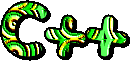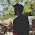## Wednesday, 12 September 2012

### Sequence & Series

Q1: Write a program to to find the sum of series as:
S = 12 + 22 + 32 + ... + n2

Answer:

```// program to find sum of series
// S = 1^2 + 3^2 + 5^2 + ... + n^2
//
#include <iostream.h>
#include <conio.h>
#include <manip.h>

int main()
{
clrscr();             // clear the screen
unsigned int n;
unsigned long sum;
clrscr();
cout << "Enter range(n) = ";
cin >> n;
sum = 0;
cout << "S = ";
for (int i = 1; i <= n; i=i+2)
{
cout << i << "^" << 2;
if (i < n)
cout << " + ";
sum += i*i;
}
cout << "\nSum = " << sum << endl;
getch();
return 0;
}
```

The program will have the following output:

Q2: Write a program to sum the following sequence?
S = x + x2/2 + x3/3 + x4/4 +... + xn/n

Answer:

```// program to sum the following sequence
// x + x^2/2 + x^3/3 + x^4/4 + ... + x^n/n
//
#include <iostream.h>
#include <conio.h>
#include <math.h>

int main()
{
clrscr();             // clear the screen
int x,n;
float sum = 0.0;
clrscr();
cout << "Enter the value of x= ";
cin >> x;
cout << "Enter the value of n= ";
cin >> n;

for (int i = 1; i <=n; ++i)
{
if (i == 1)
{
sum = x;
continue;
}
sum += ((float)pow(x,i)/(float)i);
}
cout << "Sum=" << sum;
getch();
return 0;
}
```

Q3: Write a program to find the sum of the following sequence:
S = x - x2/2! + x3/3! - x4/4! +x5/5! - x6/6!

Answer:

```// program to find sum the following sequence
// x - x^2/2! + x^3/3! - x^4/4! + x^5/5! - x^6/6!
//
#include <iostream.h>
#include <conio.h>
#include <math.h>

int main()
{
clrscr();             // clear the screen
int x, k;
double sum = 0.0;
cout << "Enter the value of x= ";
cin >> x;
k = -1;

for (int i = 1; i <=6; ++i)
{
k = k * -1;
if (i == 1)
{
sum = x;
continue;
}
// calculate factorial
double fact = 1.0;
for (int j = 1; j <= i; ++j)
{
fact *= j;
}
sum += ((double)pow(x,i)/fact)*k;
}
cout << "Sum=" << sum;
getch();
return 0;
}
```

The program will have the following output:

Q4: Write a program to print first 10 Fibonacci numbers.

Answer:

```// program to find sum the following sequence
// write a program to print first 10 Fibonacci numbers
//
#include <iostream.h>
#include <conio.h>

int main()
{
int n1, n2, n3;
n1 = 0;
n2 = 1;
// clear screen
clrscr();
for (int i = 0; i < 10; ++i)
{
// print first two fibonacci numbers
if (i == 0)
cout << n1;
else if (i == 1)
cout << n2;
else
{
// compute next fibonacci number
n3 = n1 + n2;
cout << n3;
n1 = n2;
n2 = n3;
}
cout << "  ";
}
getch();
return 0;
}
```

The program will have the following output:

Q5: Write a  c++ program to input integers in an array  of  size 10. Find the sum of even numbers in that array.

Answer:

```// wap to input an integer array of size 10
// and find the sum of even numbers in an array
//
#include <iostream.h>
#include <conio.h>

int main()
{
int arr;
int i;
clrscr();
cout << "Enter 10 integers\n";
for (i = 0; i < 10; ++i)
{
cout << "Enter number [" << i+1 << "]=";
cin >> arr[i];
}
// compute sum of even numbers
long sum = 0;
int evens = 0;
for (i = 0; i < 10; ++i)
{
// check if number is even, then add
if (arr[i] % 2 == 0)
{
sum += arr[i];
evens++;
}
}
cout << "There are " << evens << " evens and their sum=" << sum << endl;
getch();
return 0;
}
```

Q6: Write a program to find the sum of digits.
e.g. if number entered is 2374, S = 2+3+7+4 = 16

Answer:

```// program to find the sum of a number
// e.g. 123, sum = 1 + 2 + 3
//
#include <iostream.h>
#include <conio.h>

int main()
{
unsigned int num, digit;
clrscr();
cout << "Enter an integer number:";
cin >> num;
long sum = 0;
cout << "Sum of digits:" << num << "\n";
while (num > 0)
{
digit = num % 10;
sum = sum + digit;
num = num / 10;
cout << digit << "+";
}
cout << "=" << sum << endl;
getch();
return 0;
}
```

Go to Snippets-5
.#### 5 comments:

1.write a program in c++ to display a matrix of size m*n

1.This comment has been removed by the author.

2.This comment has been removed by the author.

3.Oh god damn it kitne dumb ho yaarr tum log sharam nahi aati matrices ka doubt puchte hue

4.Write to u soon

We love to hear your thoughts about this post!